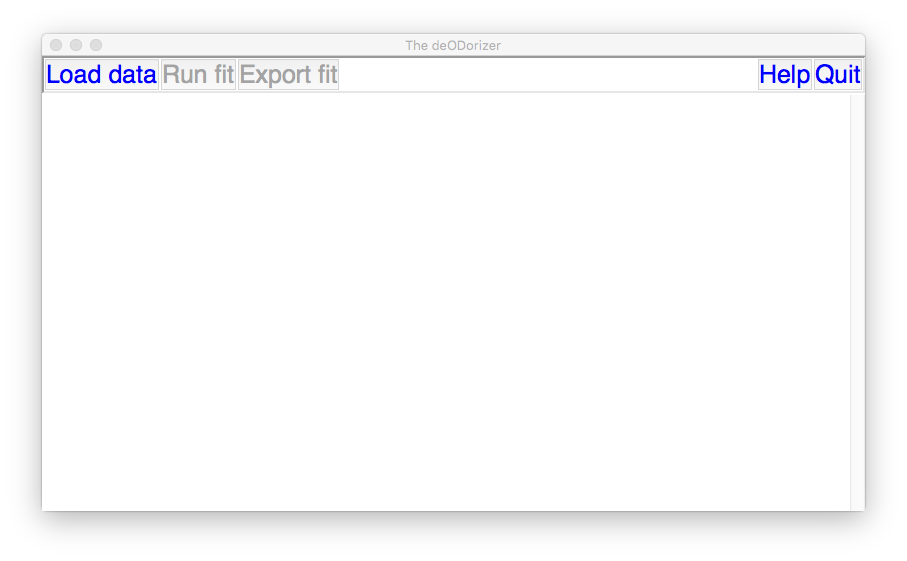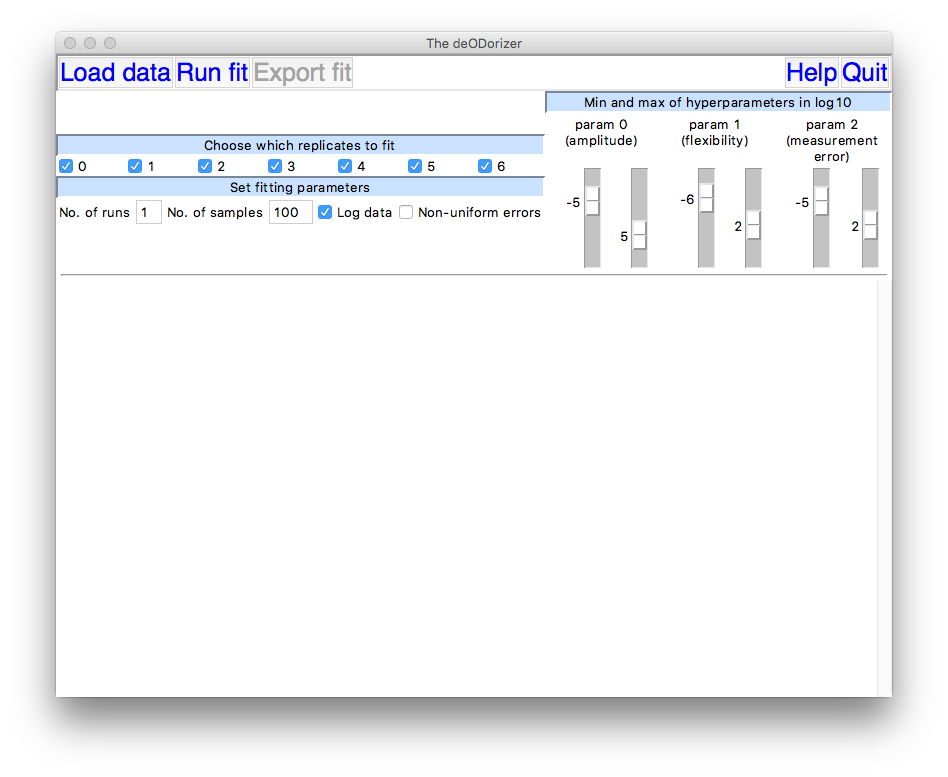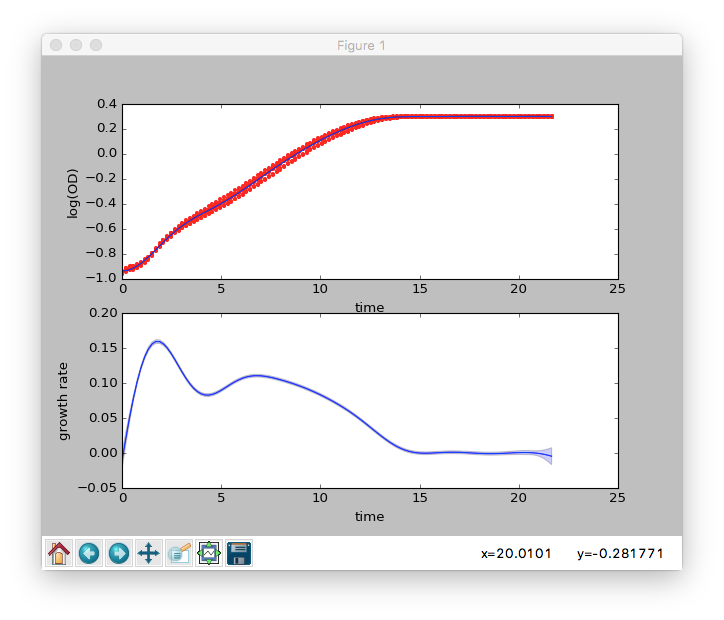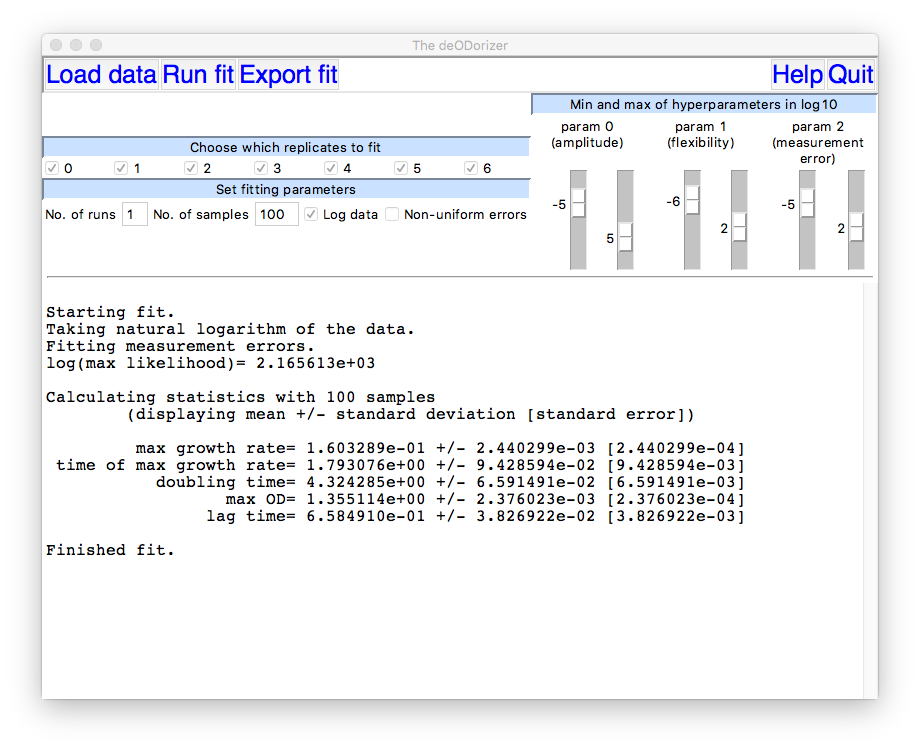Inferring time-derivatives including cell growth rates using Gaussian processes

The analysis code is written in Python 3 and all the prerequisites, including Python 3, can be installed at once using the Anaconda package. The software uses the NumPy, SciPy, Matplotlib and Pandas modules, and installing Anaconda, or an equivalently package, is much more straighforward than installing the modules individually, even if you already have Python on your computer.

You need three files: fitderiv.py, fitderivgui.py, and gaussianprocess.py, but we have also included some example data and an example script.

These files must all be placed in the same directory.

To get started, you should launch a terminal (a command prompt on Windows) and ensure that the terminal accesses this directory before starting Python (using the cd command to change to the directory).

Please let us know of any bugs or of suggested improvements at swainlabsoftware at gmail dot com.

Contents

Running the GUI
Using a script
Trouble-shooting
Acknowledging the software

Running the GUI

The GUI is written to provide a tool for estimating growth rates from optical density data and uses that language (although it can process any other type of data too):

1. Start the GUI by typing

python fitderivgui.py

in a terminal (at the command prompt).

The GUI window will take a few seconds to appear the first time it is called and should look like this on a Mac.2. Clicking on Help will produce a new window with detailed instructions.

3. Load the data by clicking on Load data. The data may be a .xlsx, a .csv, or a .txt file. The first column of data should be time and subsequent columns should be measurements of optical density (OD). The data can also be arranged in rows (i.e. the first row consists of time points, the second row consists of OD measurements, etc.).

For a data set with four replicate measurements of OD, the GUI window should now look something like this:4. Run the fit by clicking on Run fit. If you wish you can omit some replicates by de-selecting the appropriate box.

Once the fit has completed, a new window will display the results graphically. The upper subplot shows the fit to the logarithm of the OD data and the lower subplot shows the inferred growth rate. For both, the dark blue line is the best-fit and the light blue is an estimate of the error in this fit.The main GUI window will display numerical results from the fitting procedure including estimates for various summary statistics of the growth curve:By sliding the scale bars for the hyperparameters, you can change their upper and lower bounds and then re-run the fit. The lower bound for the hyperparameter for the amplitude (param 0) is here 10-5 and the upper bound is 105.

You may wish to change the bounds if one hyperparameter is optimized to lie exactly on a bound (the GUI window will display a warning) or if you dislike the smoothness of the best-fit lines (change then the bounds for the flexibility parameter).

5. You can export the results to, for example, an .xlsx file with Export fit. An additional file with the suffix _stats.xlsx in this case will be created with the summary statistics.

6. You can then either load a new data set with Load data or shut down the entire GUI with Quit.

7. Further information is available under Help.

8. Figures 2A and 2B can be recreated in the GUI. For Figure 2B, non-uniform errors should be toggled on.

Using a script

We give below an example of Python code to call the algorithm using a script where we do not rely on the default bounds for the hyperparameters but specify particular values.

import numpy as np
from fitderiv import fitderiv
import matplotlib.pyplot as plt

t, od= d[:,0], d[:, 1:]

# redefine bounds and run inference
b= {0: [-1,5], 1: [-5,2], 2: [-7,1]}
q= fitderiv(t, od, bd= b)

# plot results
plt.figure()
plt.subplot(2,1,1)
q.plotfit('f')
plt.subplot(2,1,2)
q.plotfit('df')
plt.show()

# export results
q.export('results.xlsx')

Further information can be found by typing

help(fitderiv)

in for example IPython after the module has been imported.

Troubleshooting

If there is a poor fit to the data, try decreasing the upper bound on the hyperparameter for the measurement error.

If the inferred growth rate is too "noisy", try either increasing the lower bound on the hyperparameter for the measurement error to prevent over-fitting or decreasing the upper bound on the hyperparameter for the flexibility.

If the best-fit appears to change when you re-run the fitting, then the optimization is probably finding a local optimum. Try increasing the number of runs, say to either 5 or 10.

We tend to favour the Matern covariance function and, for data from our plate readers, typically raise the lower bound on the hyperparameter for the measurement error (the last one) to prevent overfitting.